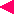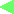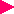Top of Part 1Last pageTop of Part 2 1-8: Summary of Part 1

 Let us summarize what we have learned in Part 1.(1)Schroedinger introduceda wave equationwhich the de Broglie waveaccompanying the motion ofa substance particleshould satisfy.It was called theSchroedinger equationand becamethe fundamental equationof quantum mechanics.(2)Heisenberg thoughtthat, in the microscopic world,the position and momentumcoordinates, qandp ,are not such ordinary variablesas those in the classical mechanicsbut that they are matrices.Thereby he proposedthe matrix mechanics.It was clarified thatthese two types of mechanics,Schroedinger's wave mechanicsandHeisenberg's matrix mechanics,are equivalentto each other.(3)It had been clarifiedin the microscopic world thatit is in principleimpossible to measurethe position and momentumof a particle more preciselythan the limitationsprescribed byHeisenberg's uncertaintyprinciple.Accordingly,it was made clear that,in the microscopic world,the concept of "orbit"of a particle,which can be describedby a curved "line",must be abandoned.(4)The probability interpretationof the wave functionwas proposed.It claims thatthe square of the absolutevalue of the wave functiondenotes the probability densitythat the particlewill be found.It was elucidatedthat the tunnel effect,based on this idea,can explain the alpha decayof atomic nuclei.This was one of the strongproofs of the validityof quantum mechanics.Go back to the top page of Part 1.Go back to the last page.Go to the top page of Part 2.